Biology is the Science Paper - 3. The duration of the exam is two hours. You have to attempt all questions from Section I and any four questions from Section II.

### Section I (40 Marks)

Question 1

(a) Name the following: 

(i) The hormone that regulates the basal metabolic rate.
(ii) The part of the internal ear related to the static balance of the body.
(iii) The soluble protein in blood plasma responsible for blood clotting.
(iv) The gaseous plant hormone.
(v) The uptake of mineral ions against the concentration gradient.

(b) Choose the correct answer from the four options given for each below: 

(i) The rate of transpiration will be fastest when the day is:
A. Hot, humid and windy
B. Cool, humid and windy
C. Hot, humid and still
D. Hot, dry and windy

(ii) Cytokinins are predominantly present in:
A. Permanent tissues
B. Meristematic tissues
C. Endodermis
D. Cortical region

(iii) A cell has five pairs of chromosomes. After mitotic division, the number of chromosomes in the daughter cells will be:
A. Five
B. Ten
C. Twenty
D. Forty

(iv) Learning is related to:
A. Cerebrum
B. Cerebellum
C. Medulla Oblongata
D. Hypothalamus

(v) The most primitive ancestor of man is:
A. Homo habilis
B. Cro- magnon
C. Neanderthal
D. Australopithecus

(c) The following paragraph is related to absorption of water from the soil. Complete the following paragraph by selecting the correct word from those given below. You may use a term only once. 

exosmosis, hypertonic, osmosis, isotonic, hypotonic, cortical, endosmosis, phloem.

Water enters the root hair from the soil by the process of (i) __________. This is because the solution in the soil is (ii) __________ whereas the cell sap in the root hair cell is (iii) __________. The water then passes through the (iv) __________ cells by cell to cell (v) __________ and reaches the xylem of the root.

(d) Give the exact location of each of the following structures: 

(i) Corpus Callosum
(iii) Acrosome
(iv) Amnion
(v) Monocytes

(e) Given below are five sets of terms. In each case arrange and rewrite each set so as to be in a logical sequence. One is done as an example for you: 

Eg: Large intestine, stomach, mouth, small intestine, oesophagus.
Ans: Mouth, oesophagus, stomach, small intestine, large intestine.

(i) Metaphase, interphase, anaphase, prophase, telophase
(ii) Vagina, sperm, uterus, oviduct, cervix
(iii) Pinna, cochlea, tympanum, ossicles, auditory canal
(iv) Posterior venacava, renal artery, aorta, renal vein, kidney
(v) Synapse, axon endings, cyton, node of Ranvier, dendrite

(f) Identify the odd term in each set and name the category to which the remaining 3 belong. 

Example: Ovary, Fallopian tube, Ureter, Uterus.
Odd term: Ureter
Category: Parts of female reproductive system.

(i) Basophil, Neutrophil, Eosinophil, Lymphocyte
(ii) Pulmonary vein, Hepatic vein, Renal vein, Post caval
(iii) Gibberellin, Auxin, Vasopressin, Abscisic acid
(iv) Wind energy, Tidal energy, Petroleum, Solar energy
(v) Plastic, Paper, Glass, Aluminium

(g) The diagram given below represents a plant growing in a glass jar. The glass jar is placed near a window. Study the diagram and answer the questions that follow: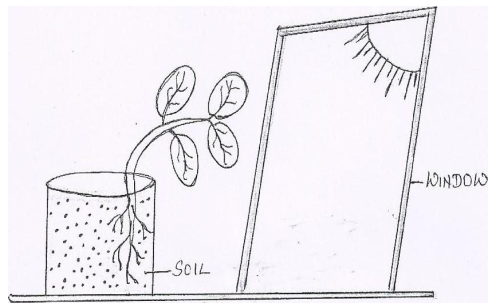(i) Name the tropic movements shown by the shoot and roots.
(ii) What is the stimulus that made the shoot bend towards the window?
(iii) Which plant hormone caused the above effect?
(iv) Explain the role of the hormone in bending the shoot towards the window.

(h) Match the items of Column A with those in Column B and rewrite the correct matching pairs: 

Column A
(i) Diabetes mellitus
(ii) Diabetes insipidus
(iii) Cretinism
(iv) Insulin shock
(v) exophthalmic goitre

Column B
– Hyper secretion of thyroxine
– Hypo secretion of thyroxine
– Hyperglycemia
– Hypo secretion of growth hormone
– Hypoglycemia

### Section II (40 Marks)

Question 2

(a) Study the diagrams given below and answer the questions that follow: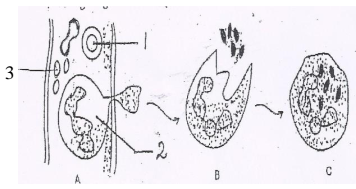(i) Name the cells labelled 1, 2 and 3.
(ii) Identify the phenomenon occurring in A. Explain the phenomenon.
(iii) Mention two structural differences between 1 and 2.
(iv) Name the process occurring in B and C.
(v) State the importance of this process in the human body.

(b) Give one difference between each of the following pairs on the basis of what is given in the brackets: 

(i) Mitral valve and Aortic semilunar valve [location]
(ii) Hydrotropism and Thigmotropism [stimulus]
(iii) Metaphase and Anaphase [position of chromatids]
(iv) Demography and Population density [definition]
(v) Turgid cell and Plasmolysed cell [tonicity of the surrounding solution]

Question 3

(a) An outline sketch of a tree is shown in the diagram below. Study the same and answer the questions that follow: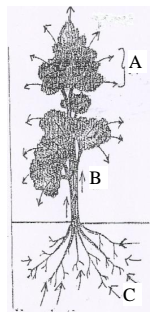(i) Name the phenomenon that is labelled A in the diagram.
(ii) Explain the phenomenon occurring in A.
(iii) What is the importance of this phenomenon in plants?
(iv) Explain the role of any three external factors that will increase the rate of the phenomenon.
(v) What do the direction of arrows in B and C indicate?

(b) Mention the exact function of the following structures: 

(i) Iris
(ii) Plasma membrane
(iii) Nephron
(iv) Thylakoids
(v) Hydathodes

Question 4

(a) Given below is a diagrammatic representation of a defect of the human eye which has been corrected using a suitable lens. Study the diagram and answer the questions that follow: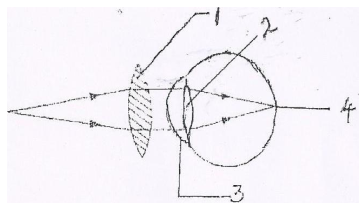(i) Identify the defect that has been corrected.
(ii) Mention two reasons for the above defect.
(iii) Label the parts numbered 1 to 4.
(iv) State the functions of the parts numbered 3 and 4.
(v) What maintains the shape of the eye ball?

(b) Give the biological / technical terms for the following: 

(i) The quick actions which are involuntary and controlled by the spinal cord.
(ii) The structure formed after the release of ovum from the Graafian follicle.
(iii) The surgical technique used in human females to prevent pregnancy.
(iv) The stage of cell division in which the nuclear membrane disappears and the Chromosomes become short and thick.
(v) The onset of menstruation in a young girl of 13 years.
(vi) The canal through which testes descend into the scrotum just before birth in a human male baby.
(vii) The repeating components of each DNA strand lengthwise.
(viii)The site of photosynthesis in a plant cell.
(ix) The constituents which cause the natural quality of the environment to deteriorate.
(x) The defect of vision in which some parts of the objects are seen in focus while the others are blurred.

Question 5

(a) The diagram below represents an experiment to demonstrate a particular aspect of a physiological process in plants. Study the diagram and answer the questions that follow: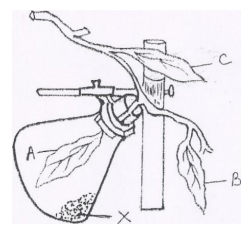(i) What is the aim of the experiment?
(ii) What is the chemical substance named X in the diagram? What is the special condition created inside the flask due to the presence of the substance X?
(iii) In what way will the three leaves A, B and C differ at the end of the experiment when tested with iodine solution?
(iv) Write the overall chemical equation for the process mentioned in (i).
(v) Explain the term 'Destarching'

(b) Briefly explain the following: 

(i) Plasmolysis
(ii) Gestation
(iii) Synapse
(iv) Photophosphorylation
(v) Lamarck's theory of use and disuse of organs

Question 6

(a) Given below is the diagram of the male reproductive system and its associated parts in the human body. Study the diagram and answer the questions that follow: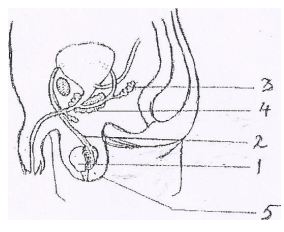(i) Name the parts labelled 3 to 5.
(ii) State the function of the part marked 2 and 3.
(iii) What is the significance of the part labelled 5.
(iv) Mention the hormone secreted by the part labelled 1.
(v) Draw a neat labelled diagram of a human sperm.

(b) Give scientific reasons for the following statements: 

(i) Foetus cannot develop without placenta.
(ii) Throat infections can lead to ear infections.
(iii) We feel blinded for a short while entering a dark room when coming from bright light.
(iv) Urine is slightly thicker in summer than in winter.
(v) Loss of nucleus and mitochondria make erythrocytes more efficient in their function.

Question 7

(a) A homozygous purple flower variety of pea plant [PP] is crossed with white flower variety of pea [pp]. Answer the questions that follow: 

(i) Mention the phenotype and genotype of the Fl generation of offsprings.
(ii) If the offsprings of the Fl generation are crossed, what will be the phenotypic and genotypic ratios of the F2 generation?
(iii) State Mendel's law of dominance.
(iv) What is the scientific name of pea plants?
(v) Name two genetic diseases in humans.

(b) Answer the following questions briefly: 

(i) Mention two features of a Neanderthal man.
(ii) What are the age restrictions for marriage by law for boys and girls in India?
(iii) State two objectives of ‘Swachh Bharat Abhiyan’.
(iv) Mention two functions of the amniotic fluid.
(v) List two reasons for the population explosion in India.